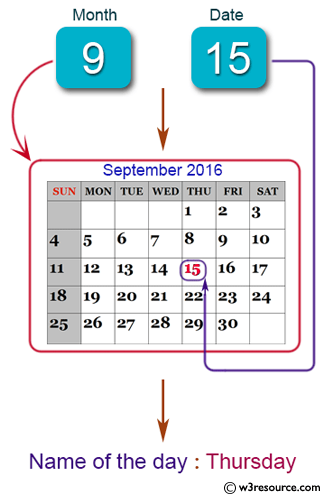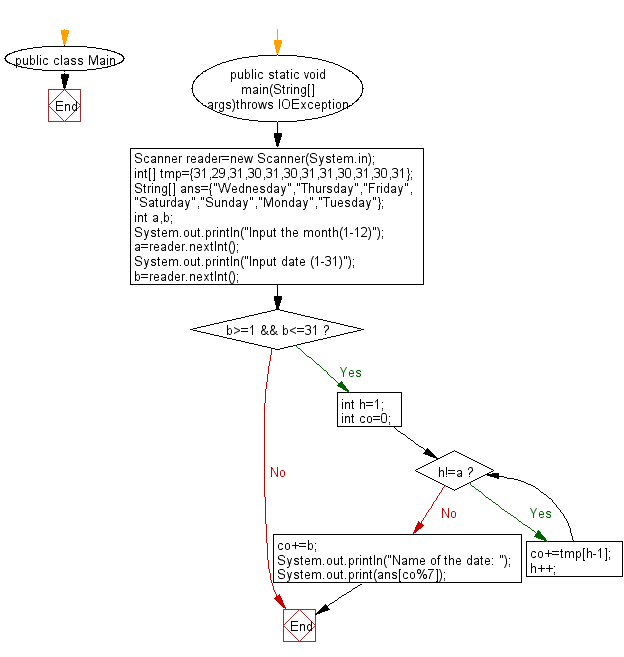# Java Exercises: Reads a date and prints the day of the date

## Java Basic: Exercise-225 with Solution

Write a Java program to that reads a date (from 2004/1/1 to 2004/12/31) and prints the day of the date. Jan. 1, 2004, is Thursday. Note that 2004 is a leap year.

Pictorial Presentation:Sample Solution:

Java Code:

``````import java.util.*;

class Main {

static int days[] = {31, 29, 31, 30, 31, 30, 31, 31, 30, 31, 30, 31};
static String name[] = {"Monday", "Tuesday", "Wednesday", "Thursday", "Friday", "Saturday", "Sunday"};

public static void main(String args[]){
Scanner in = new Scanner(System.in);
System.out.println("Input month and date(o o to exit)");

for(;;){
int m=in.nextInt(), d=in.nextInt();
if(m==0&&d==0) break;
System.out.println(solve(m,d));
}
}

static String solve(int month, int date){
int cur = 3;
System.out.println("Name of the day:");
for(int i=0; i<month-1; i++) cur += days[i];
cur += date-1;
return name[cur%7];
}

}
```
```

Sample Output:

```Input month and date(o o to exit)
9
15
Name of the day:
Wednesday
```

Flowchart:Java Code Editor:

What is the difficulty level of this exercise?

Test your Programming skills with w3resource's quiz.

﻿

## Java: Tips of the Day

Java: Anagrams

Generates all anagrams of a string.

```public static List<String> anagrams(String input) {
if (input.length() <= 2) {
return input.length() == 2
? Arrays.asList(input, input.substring(1) + input.substring(0, 1))
: Collections.singletonList(input);
}
return IntStream.range(0, input.length())
.mapToObj(i -> new SimpleEntry<>(i, input.substring(i, i + 1)))
.flatMap(entry ->
anagrams(input.substring(0, entry.getKey()) + input.substring(entry.getKey() + 1))
.stream()
.map(s -> entry.getValue() + s))
.collect(Collectors.toList());
}
```